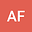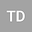Critical exponent for semi-linear structurally damped wave equation of derivative type
••## Abstract

The main purpose of this paper is to study the following semi-linear structurally damped wave equation with nonlinearity of derivative type: $$u_{tt}- \Delta u+ \mu(-\Delta)^{\sigma/2} u_t= |u_t|^p,\quad u(0,x)= u_0(x),\quad u_t(0,x)=u_1(x),$$ with $\mu>0$, $n\geq1$, $\sigma \in (0,2]$ and $p>1$. In particular, we are going to prove the non-existence of global weak solutions by using a new test function and suitable sign assumptions on the initial data in both the subcritical case and the critical case.
27 Apr 2020Submitted to Mathematical Methods in the Applied Sciences
02 May 2020Submission Checks Completed
02 May 2020Assigned to Editor
06 May 2020Reviewer(s) Assigned
31 May 2020Review(s) Completed, Editorial Evaluation Pending
01 Jun 2020Editorial Decision: Revise Minor